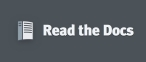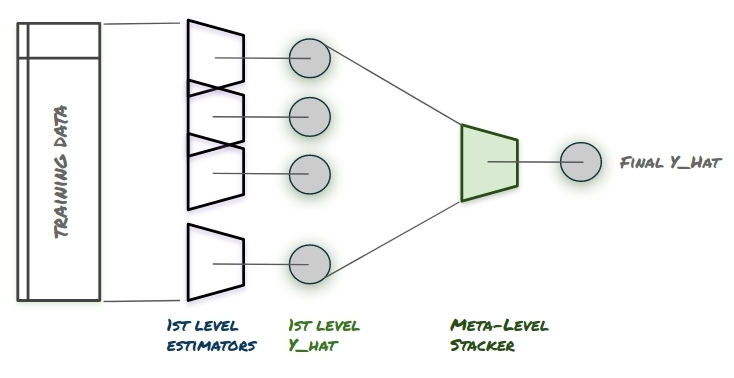# Stacker¶

Ensemble learning combining multiple regression models into a single regression pipeline

Shahar Azulay, Ariel HanemannThere are three so-called “meta-algorithms” / approaches to combine several machine learning techniques into one predictive model in order to:

1. decrease the variance (bagging)
2. decrease the bias (boosting)
3. or improving the predictive force (stacking).

Stacking is an ensemble learning method in which one applys several models to your original data at the first stage. Each of the models are trained to fit and best estimate the data in a predictive manner.

At the second stage, the models are not combined in a standard way (e.g. averaging their outputs) but rather meta-level model is introduced to estimate the input together with outputs of every model to estimate the weight each model should get or, in other words, determine which models perform better than others on the certain types of input data.high-level description of the stacking ensemble

Stacking is tricky and should be performed with care. Without a careful implementation stacking can be easily drawn into a very overfitted model, that fails to generalize well on the input data.

The Stacker module allows for easy use of the stacking apporoach in an sklearn-based environment.

First time example:

>>> from sklearn.decomposition import PCA
>>> from sklearn.pipeline import Pipeline
>>> from sklearn.tree import DecisionTreeRegressor
>>> from sklearn.linear_model import LinearRegression
>>> import stacker
>>>
>>> from sklearn.datasets import load_boston
>>> X, y = load_boston(return_X_y=True)
>>>
>>> tr, te = self._default_cv_fun().split(X).next()
>>> X_train, X_test = X[tr], X[te]
>>>
>>> my_pipe = Pipeline([
('pca', PCA()),
('dtr', DecisionTreeRegressor(random_state=1))])
>>> my_regr = LinearRegression()
>>> my_meta_regr = LinearRegression()
>>>
>>> stck = stacker.Stacker(
first_level_preds=[my_pipe, my_regr],
stacker_pred=my_meta_regr,
n_jobs=-1)
>>>
>>> stck.fit(X[tr], y[tr])
>>> y_hat = stck.predict(X[te])